Smartick is an advanced online program that teaches kids math and coding in only 15 min. a day

Mar09

# Learn about Equivalent Fractions and Practice!

### What are equivalent fractions?

Equivalent fractions are fractions that represent the same quantity, even though the numerator and denominator are different.
For example, we have two identical cakes. From one cake, we eat half a piece and from the other, we eat 2 quarters of a piece, which cake has more left?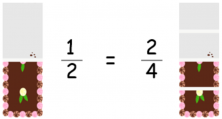Exactly! Both cakes have the same amount. Although we have represented the first one with a half and the second with two quarters, the two cakes represent the same amount. These two fractions are equivalent fractions.
Okay, we know what they are, now what?

### How can I find equivalent fractions?

There are several ways to do this. Today, I am going to show you one of them using the multiplication chart.
For example, we will find other fractions that are equivalent to a half.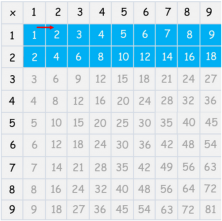Look at the table and use the first and second row, colored blue. To find an equivalent fraction of one half, we move one column to the right and have the same as before, two quarters.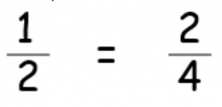The next equivalent fraction would be three-sixths if we keep moving to the right.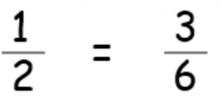And so on. Each column to the right is an equivalent fraction.

Another example:
Now we want to calculate fractions equivalent to three-fifths.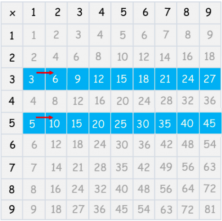We look at row 3 and row 5 and we move to the right to find equivalent fractions.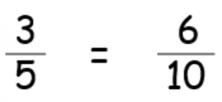Easy, right?

And at Smartick there are many more math exercises to practice! Come and try it for free.